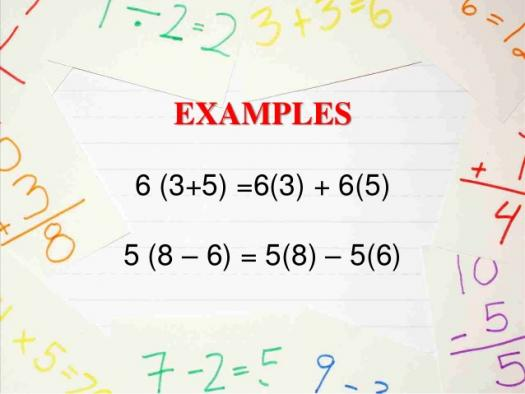# Do You Know Common Denominators?

10 Questions | Total Attempts: 113SettingsIn mathematics, the common denominator is the common multiple of the denominators of a set of fraction. It simplifies adding, subtracting, and comparing fractions.

• 1.
In ... common demoninator is the common multiple of the denominators of a set of fractions?
• A.

Mathematics

• B.

English Language

• C.

Physics

• D.

Chemistry

• 2.
The common denominator is the common multiple of the denominators of a set of ....?
• A.

Decimals

• B.

Fractions

• C.

Equations

• D.

Roots

• 3.
Common denominator is the easiest method to add or subtract ....?
• A.

Fractions

• B.

Sines

• C.

Cosines

• D.

Decimals

• 4.
The denominator is the ..... in a fraction.
• A.

Top number

• B.

Whole number

• C.

Bottom number

• D.

Total number

• 5.
When the denominators of .... fractions are the same, they are Common Denominators.
• A.

Two or more

• B.

One or two

• C.

One

• D.

None

• 6.
Common Denominator is a topic taught in ....?
• A.

Physics

• B.

Mathematics

• C.

Chemistry

• D.

Biology

• 7.
Common Denominator has to do with .....?
• A.

Numbers

• B.

Alphabets

• C.

Signs

• D.

All of the above

• 8.
Mathematics is usually taught at ...?
• A.

Church

• B.

School

• C.

Home

• D.

Mosque

• 9.
LCD is the .... of all the common denominators.
• A.

Smallest

• B.

Highest

• C.

Equal

• D.

Average

• 10.
The Least Common Multiple of 3 and 6 is ...?
• A.

4

• B.

2

• C.

6

• D.

3

Related TopicsBack to top